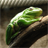# QlikView App Dev

Discussion Board for collaboration related to QlikView App Development.

Announcements
Customer & Partners, DEC. 9, 11 AM ET: Qlik Product & Strategy Roadmap Session: Data Analytics REGISTER NOW
cancel
Showing results for
Did you mean:Creator II

## Set Expression - For Rolling Quarters

Hello Everyone,

I want to write set expression wherein I have to calculate total amount for Four rolling quarters, starting with the current quarter and the values should not be affected by the selection of the quarters or years on the dashboard.

I have tried below expression, by which I am getting correct values, but when I select Quarter from the filters on the dashboard the value changes with respect to the selection, which is wrong.

((Sum({<QuarterYear=,Year=,

CloseDate = {">=\$(=Quarterstart(min(Date)))<=\$(=QuarterEnd(min(Date),3))"}>} Amount))

Please guide me to get the required output.

1 Solution

Accepted SolutionsMVP

Hi,

Try this, put all your date related fields in the set analysis like below

((Sum({<QuarterYear=,Year=, Quarter=, Month=,

CloseDate = {">=\$(=Quarterstart(date(today())))<=\$(=QuarterEnd(date(today()),3))"}>} Amount))

8 RepliesMVP

Hi,

Try like this for last 6 quarters, based on your requirement change the number in QuarterStart().

Sum({<QuarterYear=,Year=,

CloseDate = {">=\$(=Quarterstart(max(Date), -5))<=\$(=QuarterEnd(Max(Date)))"}>} Amount)

Hope this helps you.

Regards,

Jagan.Specialist II

hi try this

((Sum({<Quarter=,Date=,QuarterYear=,Year=,

CloseDate = {">=\$(=Quarterstart(min(Date)))<=\$(=QuarterEnd(min(Date),3))"}>} Amount))Creator II
Author

Hi jagan,

appreciated. When i use this expression, values still varies based on selection of quarters.

But i have try below expression and now the values are freeze and correct too.

What is your thought on this.

((Sum({<QuarterYear=,Year=,

CloseDate = {">=\$(=Quarterstart(date(today())))<=\$(=QuarterEnd(date(today()),3))"}>} Amount))MVP

Hi,

Try this, put all your date related fields in the set analysis like below

((Sum({<QuarterYear=,Year=, Quarter=, Month=,

CloseDate = {">=\$(=Quarterstart(date(today())))<=\$(=QuarterEnd(date(today()),3))"}>} Amount))Creator II

Look into using AsOfTables. Search for it in this forum, there is tons of information about it.Creator II
Author

i did the same way i wrote earlier to jagan mohan and its working

thnks muchCreator II
Author